### Basic concept of evenness

• Evenness describes how abundance is distributed across all the species in a population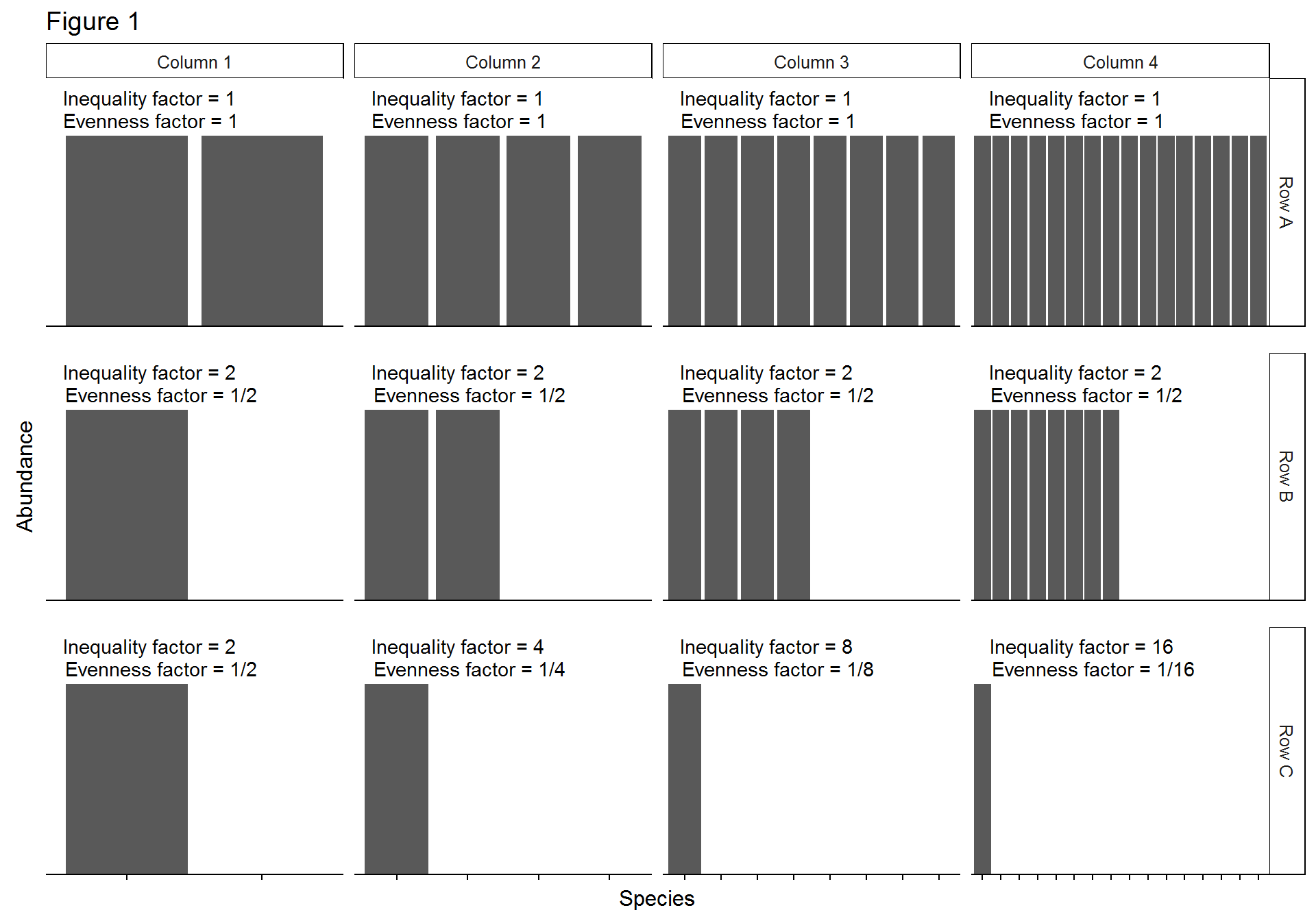• In Row A, all populations are maximally even, since every species has the same abundance
• In Row C, all populations are minimally even, since only one of all possible species has all the abundance

### Interdependence of evenness and diversity

• Diversity is a compound metric composed of both richness (number of species) and evenness
• Traditionally, it was held that richness and evenness should be defined as to be indepenendent of one another
• However, in truth, it is not possible to separate richness and evenness completely
• In the case of exponential Shannon entropy (diversity of $$q = 1$$, $$D^1$$), diversity can be decomposed to $$D^1 = e^{Shannon} = S \cdot EF_{0,1}$$
• $$S$$ - richness
• $$EF_{0,1}$$ - evenness
• $$\boxed{EF_{0,q} = D^q/D^0}$$
• Can solve for evenness to obtain $$Eveness = E_{0,1} = D^1/S$$
• $$S$$ and $$EF_{0,1}$$ are dependent because their values constrain each other
• If $$S = 2$$ (i.e. two species)
• $$D^1_{min} = 1$$ - This would be the case if one species had all the population’s abundance
• $$D^1_{max} = 2$$ - This would be the case if both species had equal abudnance
• Since $$S$$ is fixed and $$D^1$$ is bound, $$EF_{0,1}$$ must be reciprocally bound (i.e. not independent)
• In this example, $$EF_{0,1}$$ would be bound between 0.5 and 1 in order for $$S \cdot EF_{0,1}$$ to stay within the bounds of $$D^1$$

### Independence of inequality and diversity

• Alternative, richness can be decomposed into diversity and an inequality factor, $$S = D^q \cdot IF_{0,q}$$
• Proof
• $$S = D^q \cdot X$$
• $$X$$ being some factor that combines with diversity to for richness
• $$X = S/D^q$$
• $$X = D^0/D^q$$
• $$X = \boxed{IF_{0,q} = D^0/D^q}$$
• Note that $$IF_{0,q}$$ is the reciprocal of $$EF_{0,q}$$
• $$IF_{0,q}$$ and $$D^q$$ are independent because their values do not constrain each other
• If $$D^1 = 20$$
• A $$D^1$$ of $$20$$ could be obtained by an infinite number of possible populations
• $$S \ge 20$$ since $$D^0$$ (richness or $$S$$) $$\ge D^q$$
• So $$\ge 20 = (IF_{0,1})(20)$$
• Therefore $$Inf > IF_{0,1} > 1$$
• Since, by definition, $$IF_{0,q}$$ is already bound by infinity and one, the fixed value of $$D^q$$ imposed no constraint

### Interpretation of evenness and inequality

In terms of frequency

• $$D^1$$ is approximately equal to the number of common species and $$D^2$$ is approximately equal to the number of abundant species
• Thus $$D^2/S$$, for example, is approximately equal to the proportion of abundant species
• Since $$D^2/S = D^2/D^0 = EF_{0,2}$$, $$EF_{0,2}$$ is also approximately equal to the proportion of abundant species
• Thus $$EF_{0,q}$$ is approximately equal to the proportion of species represented by $$q$$
• As a corollary, $$1-EF_{0,q}$$ is the proportion of rare species not included by $$q$$

In terms of “maximally uneven” communities

• As with diversity, there are numerous population configuration that can generate the same evenness value
• Every evenness value will be equivalent to one maximmally uneven population with a specific number of species
• For a maximally uneven species, $$EF_{0,1} = D^1/S = 1/S$$
• $$D^1$$ approaches $$1$$ when all the population belongs to one species
• Thus, $$EF_{0,1}$$ can be interpreted as (the number of species in an equivalent maximally uneven community)$$^{-1}$$
• E.g. If $$EF_{0,1} = 0.125$$, then $$S = 8$$
• $$S = 1/EF_{0,1} = 1/0.125 = 8$$
• So a population with $$EF_{0,1} = 0.125$$ is as even as a maximally uneven population with $$8$$ species
• In otherwords, a population with $$EF_{0,1} = 0.125$$ is as even as the population depicted in Figure 1, row C, column A
• Inequality is simply equal to the number of species in a maximally uneven population with the same inequality value
• $$IF_{0,1} = S/D^1 = S/1 = S$$
In terms of diversity plots
• A plot of a population’s diversity values at various orders is a diversity profile
• Recall that $$IF_{0,q} = D^0/D^q$$
• Therefore, $$IF_{0,q}$$ is the ratio of the heigh of the diversity profile at $$q = 0$$ to the height at $$q = q$$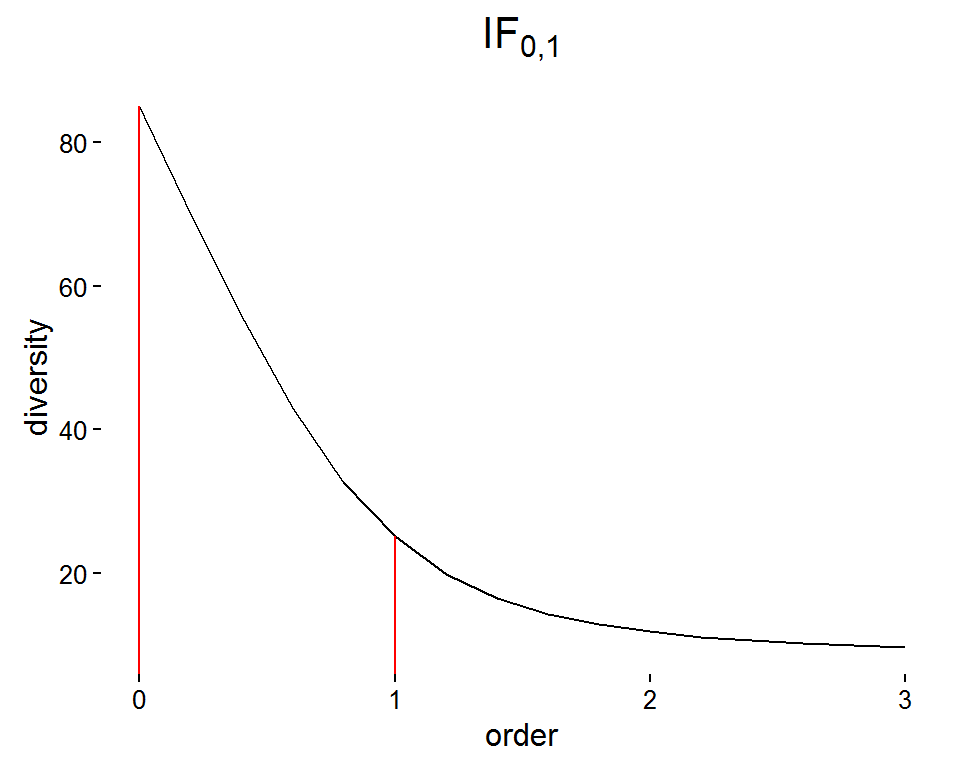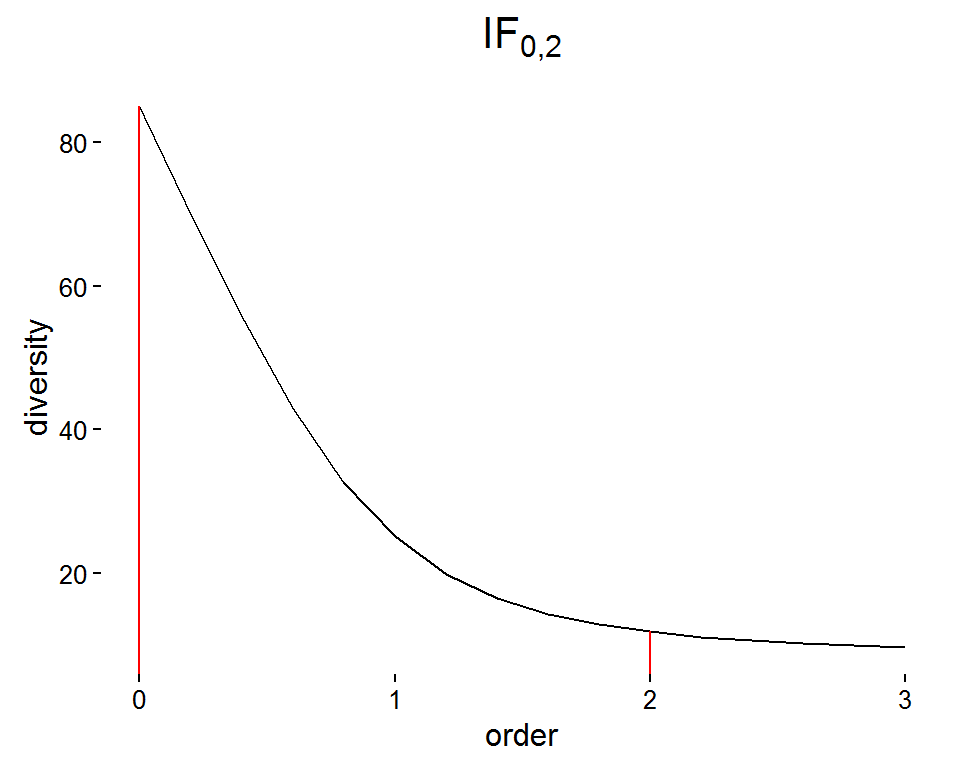• The ratio of heights for the two red lines are representations of $$IF$$
• This ratio, in turn, is simply a measure of how steeply the diversity profile decreases

In terms of mean deviation from equiprobability

• In a perfectly even community, each species is equally abundant and the frequency of of each population is $$p = 1/S$$
• In an uneven community, the deviation from perfectly even for each INDIVIDUAL can be quantified as $$p_i/\frac{1}{S}$$
• This is the proportion of the actual frequency to the perfectly even frequency
• By averaging the deviation from perfectly even for each INDIVIDUAL, the evenness of the community can be represented
• $$IF_{0,1}$$ is the geometric mean of the deviations from perfectly even for each individual
• The geometric mean is the $$n^{th}$$ root of the product of $$n$$ values, or $$(\prod_{i}^{N}x_i)^{1/N}$$
library(vegan)
pop <- c(8, 1, 1)
freq <- pop/sum(pop)
perfect.freq <- 1/(length(pop))
deviation <- freq/perfect.freq
geo.mean <- prod(deviation^pop)^(1/sum(pop))
hill <- exp(renyi(pop, scales = c(0,1)))
D0 <- hill
D1 <- hill
IF0.1 <- D0/D1
data.frame("geo mean deviation" = geo.mean, "IF0.1" = IF0.1)
##   geo.mean.deviation    IF0.1
## 0           1.583409 1.583409
• $$IF_{0,2}$$ is the arithmetic mean of the deviations from perfectly even for each species

library(vegan)
pop <- c(8, 1, 1)
freq <- pop/sum(pop)
perfect.freq <- 1/(length(pop))
deviation <- freq/perfect.freq
arith.mean <- sum(deviation * pop)/(sum(pop))
hill <- exp(renyi(pop, scales = c(0,2)))
D0 <- hill
D2 <- hill
IF0.2 <- D0/D2
data.frame("arith mean deviation" = arith.mean, "IF0.2" = IF0.2)
##   arith.mean.deviation IF0.2
## 0                 1.98  1.98

### Improved measures of evenness/inequality with monotonic transformation

• In current form, $$IF_{0,q}$$ has a minimum value of $$1$$, whereas a minimum value of $$0$$ would make more intuitive sense for “no inequality”

Logarithmic transformation

• A logarithmic transformation of $$IF_{0,q}$$ will convert the minimum value to $$0$$ (perfectly even) with an unlimited maximum value (maximally uneven)
• The log transform of $$IF_{0,1}$$ is the Theil entropy inequality
• Theil entropy is originally an economic metric of inequality between “households” or “firms”
• So, $$\boxed{ln(IF_{0,1}) = ln(D^0/D^1) = ln(S) - H} = TEI$$
• Since $$EF_{0,1}$$ is the reciprocal, $$\boxed{ln(EF_{0,1}) = ln(D^1/D^0) = H - ln(S) = -ln(IF_{0,1})} = -TEI$$

Deformed logarithmic transformation

• The deformed logarithm, or q-logarithm, is a modified log transform
• $$ln_q(X) = (X^{1-q} - 1)/(1-q)$$
• $$ln_q(EF_{0,q}) = (-q)\cdot GEI$$
• $$GEI$$ is the generalized entropy index, a well known economic index of inequality
• $$GEI = (\frac{1}{S})(\frac{1}{q(q-1)})\sum_i^S(\frac{N_i}{\mu})^q - 1$$

### The problematic effect of richness on $$IF$$ and $$EF$$

• With two maximally uneven populations, the population with more species (greater richness) will have a higher $$IF_{0,q}$$
• This is a problem because it allows for a very rich population with modest inequality to have a larger $$IF_{0,q}$$ than a low richness population that is maximally uneven
• For example, in Figure 1, the populations in (Row C - Column 1) has the same inequality as the population in (Row B - Column 4), despite the fact that the former is maximally uneven and the latter is not

Forest example

• The Jack Pine forest is a small, highly uneven population
• The Barro Colorado Island rain forest is a larger, more even population
library(vegan)
library(ggplot2)

jack.pine <- c(980, 10, 5)
jack.pine.freq <- data.frame("species" = 1:length(jack.pine), "freq" = jack.pine/sum(jack.pine))
ggplot(data = jack.pine.freq, aes(x = species, y = freq)) + geom_path() + theme_classic()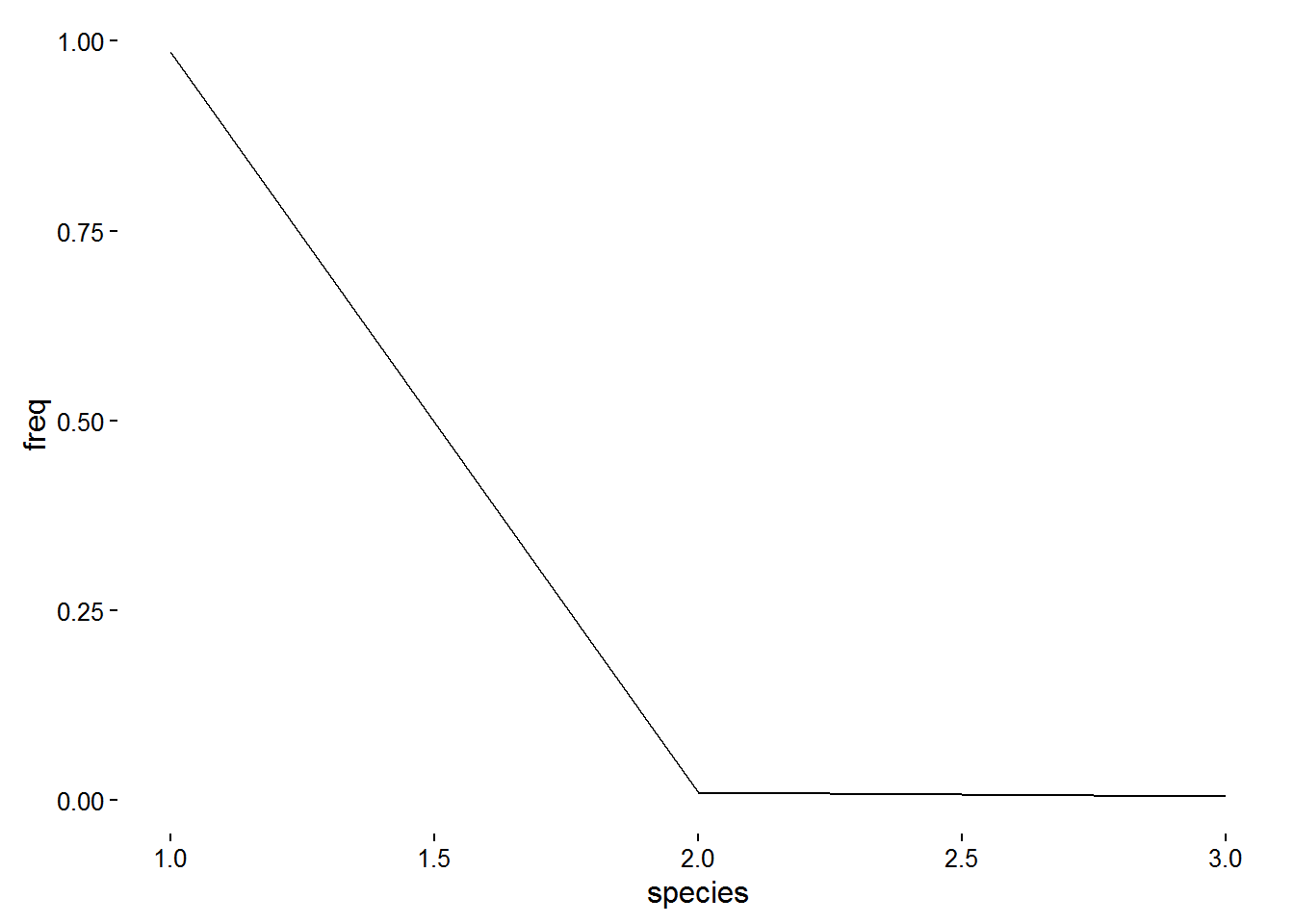hill <- exp(renyi(jack.pine, scales = c(0,1,2)))
D0 <- hill
D1 <- hill
D2 <- hill
IF0.1 <- D0/D1
IF0.2 <- D0/D2
EF0.1 <- 1/IF0.1
EF0.2 <- 1/IF0.2
data.frame(IF0.1, IF0.2, EF0.1, EF0.2)
##     IF0.1    IF0.2     EF0.1     EF0.2
## 0 2.74785 2.910608 0.3639209 0.3435708
data(BCI)
ggplot(data = barro.colorado.freq, aes(x = species, y = freq)) + geom_path() + theme_classic()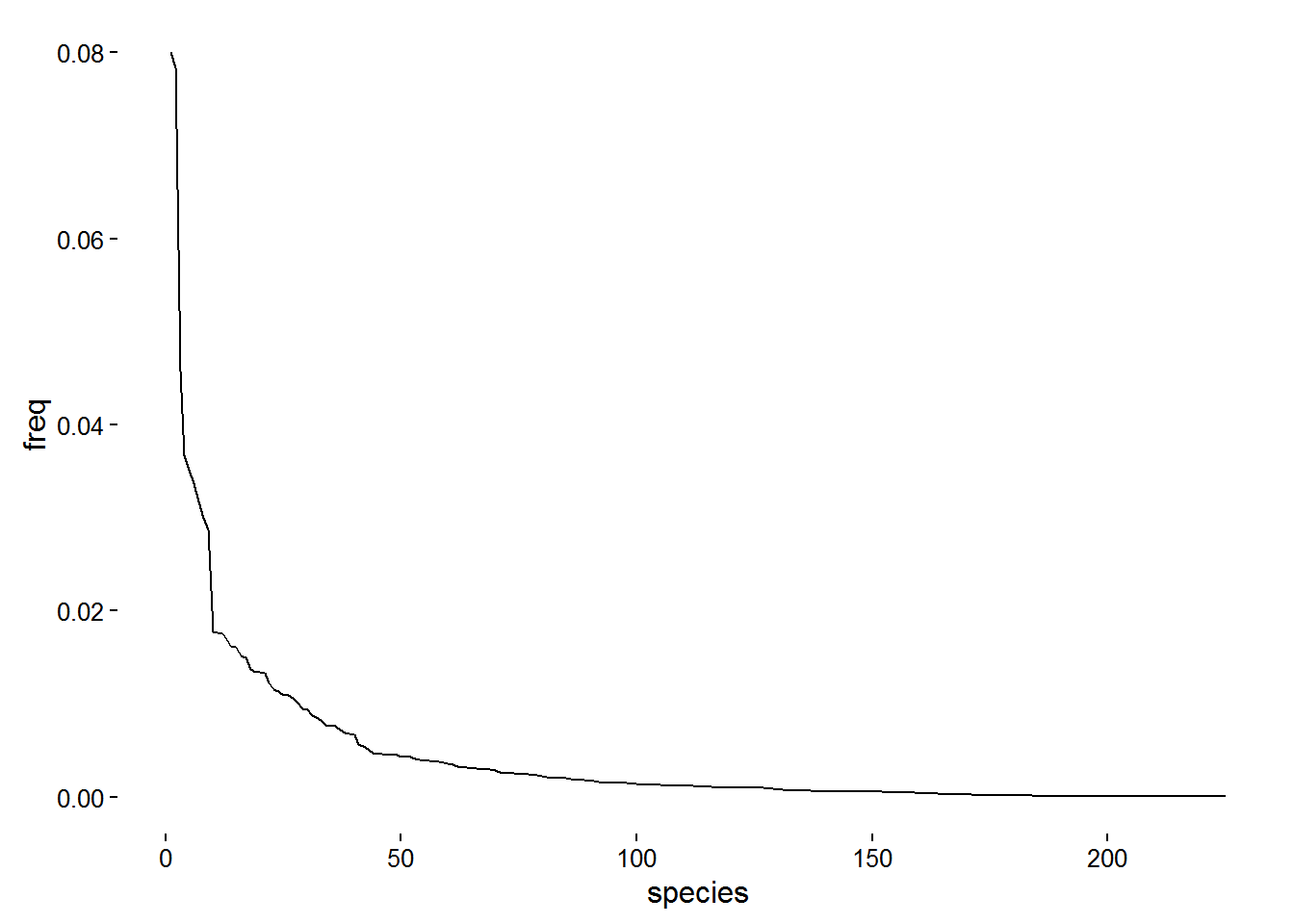hill <- exp(renyi(barro.colorado, scales = c(0,1,2)))
D0 <- hill
D1 <- hill
D2 <- hill
IF0.1 <- D0/D1
IF0.2 <- D0/D2
EF0.1 <- 1/IF0.1
EF0.2 <- 1/IF0.2
data.frame(IF0.1, IF0.2, EF0.1, EF0.2)
##      IF0.1    IF0.2     EF0.1     EF0.2
## 0 3.144616 5.923004 0.3180039 0.1688333
• Notice that for the Jack Pine forest, one species makes up nearly 100% of all individuals, whereas in the Barro Colorado rainforest, no species makes up more than 8% of all individuals
• Interpretations of evenness values
• By $$IF_{0,1}$$, the Jack Pine forest is as uneven as a maximally uneven population of 2.74785 species, whereas the Barro Colorado is equivalent to one of 3.144616 species
• Can see the validity of this as the Jack Pine forest is nearly maximally uneven and has 3 species, close to the $$IF_{0,1}$$ of 2.7
• By $$EF_{0,2}$$, in the Jack Pine forest, 34.357% of all species are “most abundant”, whereas in the Barro Colorado, only 16.88% of all species are
• Again, makes sense for the Jack Pine forest, as one out of three (33%) species dominates, close to the $$EF_{0,2}$$ of 34.357%
• Problem: Since the Jack Pine forest is nearly a maximally uneven population, its unevenness should be greater than the Barro Colorado rainforest, which is not as near to a maximally uneven population. However, by $$EF$$ and $$IF$$, the Barro Coloardo rainforest is the more uneven community as $$IF_{0,1}^{Barro}=3.14>IF_{0,1}^{Jack}=2.75$$
• This is because the richness of the Barro Colorado rainforest is much greater than that of the Jack Pine forest (see plot x-axis values for total species numbers)
• This problem can be addressed using relative values of evenness

### Improved measures of evenness/inequality with relative values

• As mentioned above, the way we know the Jack Pine forest is actually less even than the Barro Colorado rainforest, despite values of $$EF$$ and $$IF$$ is due to how close the Jack pine forest is to a maximally uneven population
• This suggests that the relative closeness to a maximally uneven community may help compensate for differences in richness

Linear transformation

• Linear transformation is a simple way to create a relative index
• Linear transformation = $$(x-x_{min})(x_{max} - x_{min})$$
• Applied to $$EF_{0,q}$$, this gives the relative eveness index $$RE_{0,q} = (D^q - 1)(S - 1)$$
• Derivation
• $$RE_{0,q} = (EF_{0,q}-EF_{0,q}{}_{min})(EF_{0,q}{}_{max} - EF_{0,q}{}_{min})$$
• $$RE_{0,q} = (EF_{0,q} - 1/S)(1-1/S)$$
• $$EF_{0,q}{}_{min} = 1/S$$ - since $$D^q$$ approaches $$1$$ toward maximum unevenness
• $$EF_{0,q}{}_{max} = 1$$ since at complete evenness, $$D$$ is constant at all $$q$$
• $$RE_{0,q} = S \cdot EF_{0,q} - 1)(S - 1)$$
• $$RE_{0,q} = (D^q - 1)(S - 1)$$
• $$RE_{0,q}$$ ranges from 0 (completely uneven) to 1 (completely even)
• Similarly, relative inequality $$RI_{0,q} = (IF_{0,q} - 1)(S - 1)$$
• $$RI_{0,q}$$ ranges from 0 (completely even) to 1 (completely uneven)
• Advantages of simple linear transformation
• Evenness and inequality are now relative to maximally uneven population for a given S
• Minimum values are now equal to zero
• Shortcomings of simple linear transformation
• Example

A <- data.frame("species" = 1:4, "abundance" = c(4000,1,1,1), "population" = rep("A", 4))
B <- data.frame("species" = 1:4, "abundance" = c(2000,2000,1,1), "population" = rep("B", 4))
C <- data.frame("species" = 1:4, "abundance" = c(1000,1000,1000,1000), "population" = rep("C", 4))
df <- rbind(A,B,C)
ggplot(data = df, aes(x = species, y = abundance)) + geom_bar(stat = "identity") + facet_grid(. ~ population) + theme_classic()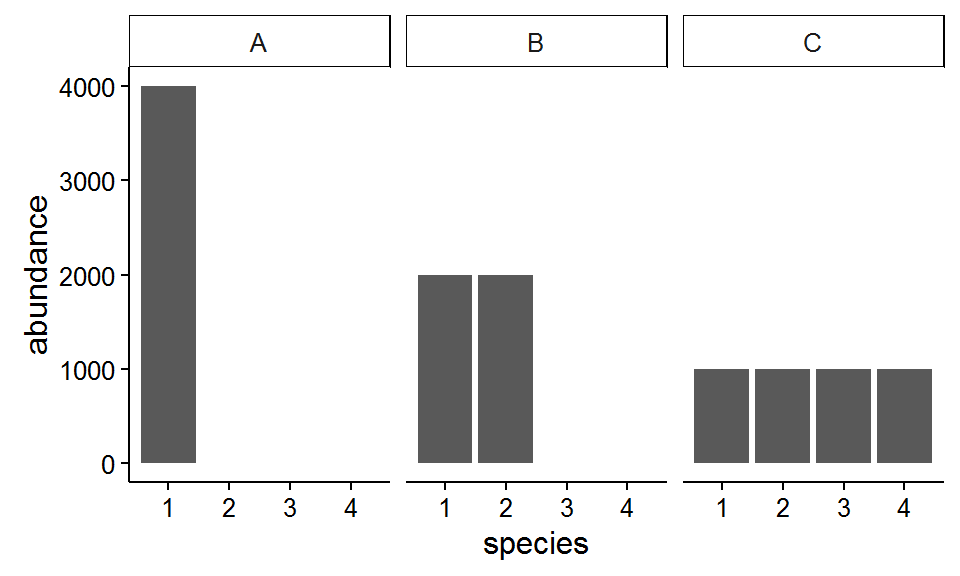IF.A <- round(as.numeric(renyi(A$abundance, hill = TRUE, scales = 0) / renyi(A$abundance, hill = TRUE, scales = 1)))
IF.B <- round(as.numeric(renyi(B$abundance, hill = TRUE, scales = 0) / renyi(B$abundance, hill = TRUE, scales = 1)))
IF.C <- round(as.numeric(renyi(C$abundance, hill = TRUE, scales = 0) / renyi(C$abundance, hill = TRUE, scales = 1)))

EF.A <- 1/IF.A
EF.B <- 1/IF.B
EF.C <- 1/IF.C

RI.A <- (IF.A - 1)/(length(A$abundance) - 1) RI.B <- (IF.B - 1)/(length(B$abundance) - 1)
RI.C <- (IF.C - 1)/(length(B$abundance) - 1) RE.A <- (length(A$abundance)*EF.A - 1)/(length(A$abundance) - 1) RE.B <- (length(B$abundance)*EF.B - 1)/(length(B$abundance) - 1) RE.C <- (length(C$abundance)*EF.C - 1)/(length(B$abundance) - 1) data.frame("population" = c("A", "B", "C"), "EF" = c(EF.A, EF.B, EF.C), "IF" = c(IF.A, IF.B, IF.C), "RE" = c(RE.A, RE.B, RE.C), "RI" = c(RI.A, RI.B, RI.C)) ## population EF IF RE RI ## 1 A 0.25 4 0.0000000 1.0000000 ## 2 B 0.50 2 0.3333333 0.3333333 ## 3 C 1.00 1 1.0000000 0.0000000 • The linear relationship between evenness and inequality as opposites is not preserved • $$EF_{0,q} \cong 1/IF_{0,q}$$ • $$RE_{0,q} \ncong 1/RI_{0,q}$$ • Notice in example how $$EF$$ and $$IF$$ are always reciprocal, where $$RE$$ and $$RI$$ are not • Particularly obvious for poulation B where $$RE = RI$$ • Relative evenness and relative inequality can be equal at a non-unity (i.e. 1) value • Makes no intuitive sense for a population to be as even as it is uneven. • Population B is an example • Transition from uneven to even is unintuitive • Population A $$\rightarrow$$ Population B involves transfer of half of population’s abundance • 100% was in species 1, 50% now in species 1 and 50% in species 2. Thus, 50% of abundance stayed with the original species (species 1) and 50% transfered, so half of the population’s abundance was transfered • Similarly, Population B $$\rightarrow$$ Population C also involves transfer of half of population’s abundance • So, Population B should be the direct intermediate of populations A and B • Since $$RE_A = 0$$ and $$RE_C = 1$$, this should make $$RE_B = 0.5$$, but this is not the case as shown in the example • In summary, the relative evenness and inequality resulting from a simple linear transformation lacks complementarity Relative logarithmic transformation • The lack of complementarity can be fixed by applying a logarithmic transform before the linear transform • Applied to $$EF_{0,q}$$, this gives the relative eveness index $$\boxed{RLE_{0,q} = ln(D^q)/ln(S)}$$ • Derivation • $$RLE_{0,q} = [ln(EF_{0,q})-ln(EF_{0,q}{}_{min})][(ln(EF_{0,q}{}_{max}) - ln(EF_{0,q}{}_{min})]$$ • $$RLE_{0,q} = [ln(EF_{0,q})-ln(1/S)][(ln(1) - ln(1/S)]$$ • $$EF_{0,q}{}_{min} = 1/S$$ - since $$D^q$$ approaches $$1$$ toward maximum unevenness • $$EF_{0,q}{}_{max} = 1$$ since() at complete evenness, $$D$$ is constant at all $$q$$ • $$RLE_{0,q} = [ln(EF_{0,q}) + ln(S)]/ln(S))$$ • $$ln(1/S) = -ln(S)$$ • $$RLE_{0,q} = [ln(D^q) - ln(S) + ln(S)]/ln(S)$$ • $$RLE_{0,q} = ln(D^q)/ln(S)$$ • Similarly, relative logarithmic inequality $$\boxed{RLI_{0,q} = ln(IF_{0,q})/ln(S)}$$ • $$RLE$$ and $$RLI$$ are reciprocal, $$\boxed{RLI = 1 - RLE}$$ • Proof 1. $$ln(IF_{0,q}) = ln(D^0/D^q)$$ as shown in log transform section 2. $$RLI_{0,q} = \frac{ln(D^0/D^q)}{ln(S)}$$ substituting (1) into $$RLI$$ equation 3. $$RLI_{0,q} = \frac{ln(S/D^q)}{ln(S)}$$ since $$D^0$$ is $$S$$ 4. $$RLI_{0,q} = \frac{ln(S) - ln(D^q)}{ln(S)}$$ 5. $$RLI_{0,q} = \frac{ln(S)}{ln(S)} - \frac{ln(D^q)}{ln(S)}$$ 6. $$RLI = 1 - RLE$$ by substitution $$RLE$$ equation in • As applied to the previous example: RLE.A <- round(as.numeric(log(renyi(A$abundance, hill = TRUE, scales = 1))/log(renyi(A$abundance, hill = TRUE, scales = 0)))) RLE.B <- round(as.numeric(log(renyi(B$abundance, hill = TRUE, scales = 1))/log(renyi(B$abundance, hill = TRUE, scales = 0)))) RLE.C <- round(as.numeric(log(renyi(C$abundance, hill = TRUE, scales = 1))/log(renyi(C$abundance, hill = TRUE, scales = 0)))) RLI.A <- log(IF.A)/log(round(as.numeric(renyi(A$abundance, hill = TRUE, scales = 0))))
RLI.B <- log(IF.B)/log(round(as.numeric(renyi(B$abundance, hill = TRUE, scales = 0)))) RLI.C <- log(IF.C)/log(round(as.numeric(renyi(C$abundance, hill = TRUE, scales = 0))))

data.frame("population" = c("A", "B", "C"),
"EF" = c(EF.A, EF.B, EF.C),
"IF" = c(IF.A, IF.B, IF.C),
"RE" = c(RE.A, RE.B, RE.C),
"RI" = c(RI.A, RI.B, RI.C),
"RLE" = c(RLE.A, RLE.B, RLE.C),
"RLI" = c(RLI.A, RLI.B, RLI.C))
##   population   EF IF        RE        RI RLE RLI
## 1          A 0.25  4 0.0000000 1.0000000   0 1.0
## 2          B 0.50  2 0.3333333 0.3333333   1 0.5
## 3          C 1.00  1 1.0000000 0.0000000   1 0.0
• Pielou’s evenness index is equivalent to $$RLE$$ at $$q = 1$$

• Recall how $$EF$$ and $$IF$$ erroneously suggest that the Jack Pine forest is more even than the Barro Colorado rainforest

hill <- exp(renyi(jack.pine, scales = c(0,1)))
D0 <- hill
D1 <- hill
IF0.1 <- D0/D1
EF0.1 <- 1/IF0.1
RLE0.1 <- log(D1)/log(D0)
RLI0.1 <- 1-RLE0.1
jp <- c(EF0.1, IF0.1, RLE0.1, RLI0.1)

hill <- exp(renyi(barro.colorado, scales = c(0,1)))
D0 <- hill
D1 <- hill
IF0.1 <- D0/D1
EF0.1 <- 1/IF0.1
RLE0.1 <- log(D1)/log(D0)
RLI0.1 <- 1-RLE0.1
bc <- c(EF0.1, IF0.1, RLE0.1, RLI0.1)

t(data.frame("Jack Pine" = jp, "Barro Colorado" = bc, row.names = c("EF0.1", "IF0.1", "RLE0.1", "RLI0.1")))
##                    EF0.1    IF0.1     RLE0.1    RLI0.1
## Jack.Pine      0.3639209 2.747850 0.07991302 0.9200870
## Barro.Colorado 0.3180039 3.144616 0.78846558 0.2115344
• Notice how $$RLE$$ and $$RLI$$ accurately represent the Jack Pine as less even than the Barrow Colorado

### Graphical interpretation of $$RLE$$ and $$RLI$$

Diversity profile shape

• If a population is replicated $$m$$-times, each point on it’s diversity profile (see earlier) is multiplied by $$m$$ as well
• This increases the steepness of the diversity profile curve
• Thus, the shape of the a diversity profile is replication dependent, which makes it difficult to compare the evenness of populations with different diversities
• The Renyi entropy spectrum is the logarithm of the diversity profile and the spectrum’s shape is replication indepenent
• Replicating a population $$m$$-times translates each point of the Renyi spectrum up by $$ln(m)$$, thus maintaining the overall shape of the spectrum
• Thus, the Renyi entropy curve is useful for comparing the eveness of populations with different diversities
pop.1 <- c(250,25,10,10,5,1)
pop.2 <- rep(pop.1/2, 2) #replicated 2 times
pop.3 <- rep(pop.1/3, 3) #replicated 3 times
pop.4 <- rep(pop.1/4, 4) #replicated 4 times
pop.5 <- rep(pop.1/5, 5) #replicated 5 times
pop.series <- list(pop.1, pop.2, pop.3, pop.4, pop.5)

hill <- vector()
renyi <- vector()
for (i in 1:5){
hill <- c(hill, renyi(pop.series[[i]], scales = seq(from = 0, to = 2, by = 0.2), hill = T))
renyi <- c(renyi, renyi(pop.series[[i]], scales = seq(from = 0, to = 2, by = 0.2)))
}

df <- data.frame("order" = rep(seq(from = 0, to = 2, by = 0.2), 10),"profile" = c(rep("diversity", 55), rep("renyi", 55)),"population" = rep(c(rep("original",11), rep("replicated (2x)", 11), rep("replicated (3x)", 11), rep("replicated (4x)", 11), rep("replicated (5x)", 11)),2),"value" = c(hill, renyi))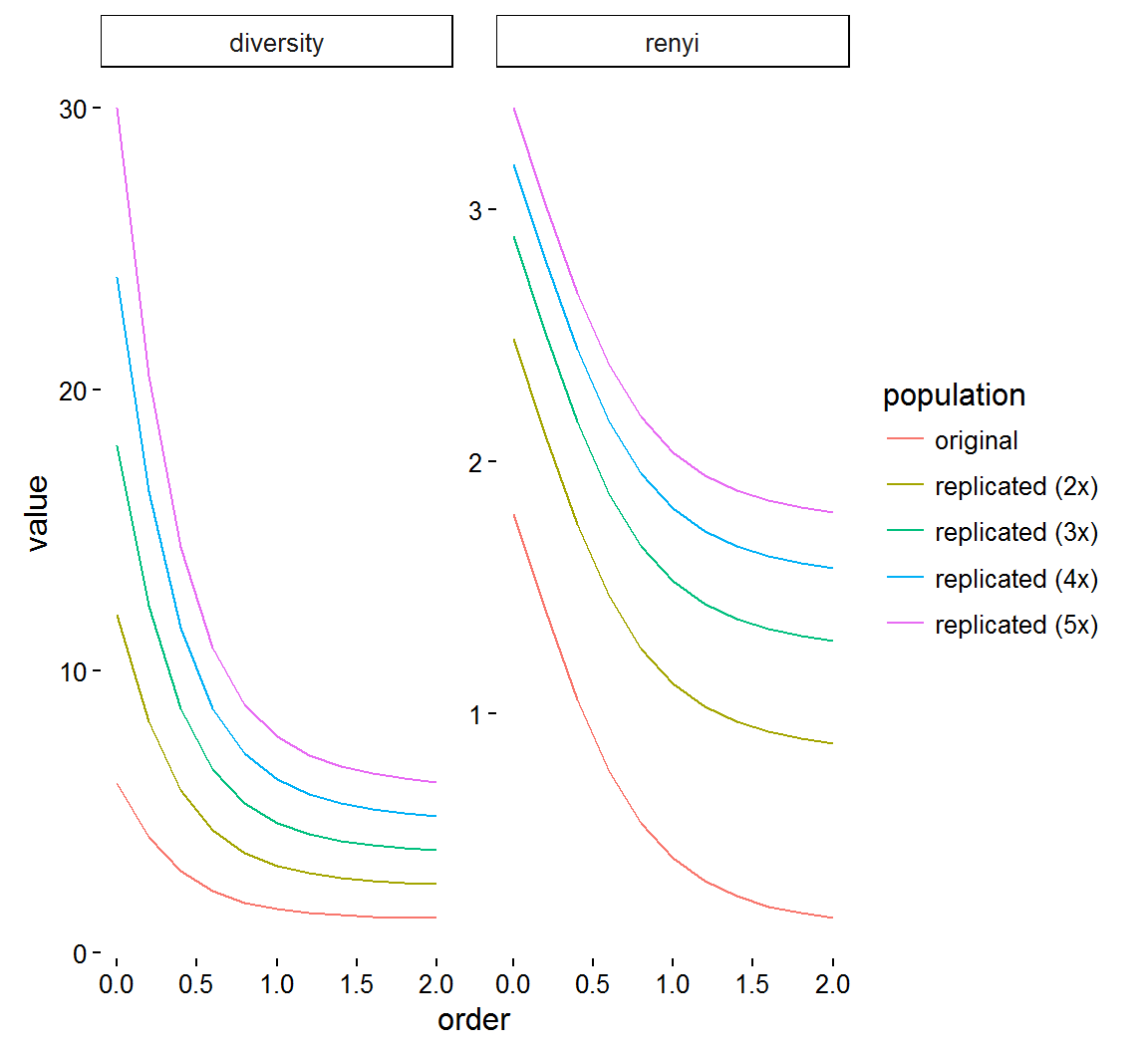• Notice how the overall shape of the diversity curve changes with replication, while the renyi profile shapes remain the same, just shifted

Relative inequality as a Renyi diversity profile chord slope

• On a Renyi spectrum curve, a chord can be drawn from $$x = 0$$ to $$x = q$$ with $$slope = \frac{\Delta y}{\Delta x} = \frac{ln(D^q - D^0)}{q} = \frac{ln(D^q/D^0)}{q} = \frac{ln(EF_{0,q})}{q}$$
• The slope can be transfored to a relative value using a linear transform again ($$(x-x_{min})(x_{max} - x_{min})$$)
• $$Slope_{max} = -ln(S)/q$$ since in a maximally uneven community (i.e. max slope), $$D^q = 1$$ so $$\frac{ln(D^q) - ln(D^0)}{q} = -ln(S)/q$$
• $$Slope_{min} = 0$$ in a perfectly even community
• So the transform of the slope is:
• $$=(\frac{ln(D^q) - ln(D^0)}{q} - 0)(\frac{-ln(S)}{q} - 0)$$
• $$=\frac{[ln(D^q) - ln(D^0)]q}{-ln(S)}$$
• $$=RLI$$
• So $$RLI$$ can be interpreted as the steepness of the slope between two Renyi entropy spectrum points relative to that of the equivalent maximally uneven population
• This means Pielou’s entropy is the reciprocal of this graphical representation
x <- c(500,300,100,50,25,25)
# x <- c(1000,1,1,1,1,1)
df <- data.frame("order" = seq(from = 0, to = 2, by = 0.2), "renyi" = renyi(x, scales = seq(from = 0, to = 2, by = 0.2)))
ggplot(data = df, aes(x = order, y = renyi)) +
geom_line() +
geom_segment(x = 0, y = df$renyi, xend = 1, yend = df$renyi, color = "red") +
theme_classic() +
ggtitle("Example population") +
theme(plot.title = element_text(size = 16)) +
annotate("text", size = 5, x = 1, y = 1.56, label = "Slope = -0.519504")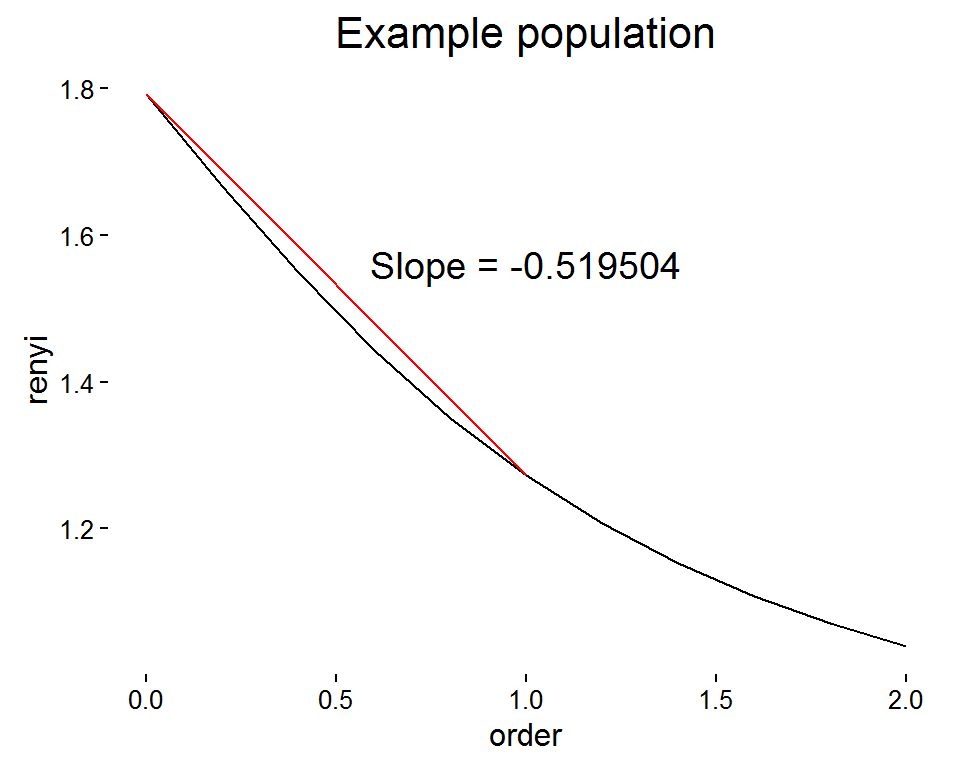# x <- c(500,300,100,50,25,25)
x <- c(1000,1,1,1,1,1)
df <- data.frame("order" = seq(from = 0, to = 2, by = 0.2), "renyi" = renyi(x, scales = seq(from = 0, to = 2, by = 0.2)))
ggplot(data = df, aes(x = order, y = renyi)) +
geom_line() +
geom_segment(x = 0, y = df$renyi, xend = 1, yend = df$renyi, color = "blue") +
theme_classic() +
ggtitle("Maximally uneven equivalent") +
theme(plot.title = element_text(size = 16)) +
annotate("text", size = 5, x = 1, y = 1.25, label = "Slope = -1.752405")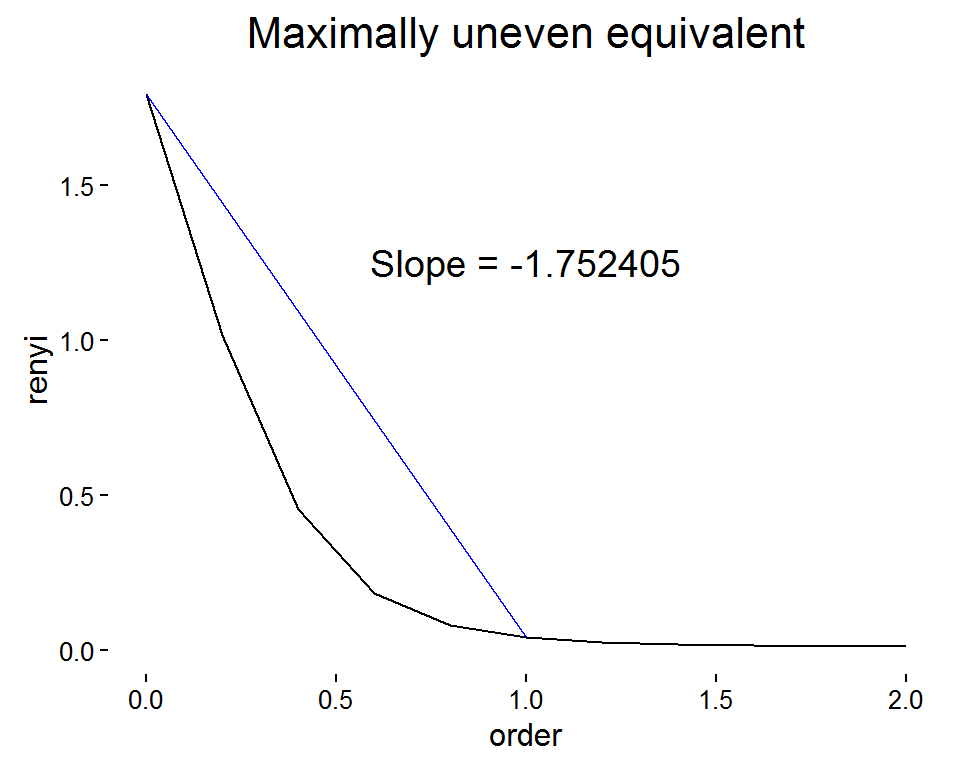• Graphically, $$RLI_{0,1}$$ is the ratio of the red slope to the blue slope

x <- c(500,300,100,50,25,25)
r <- renyi(x, scales = c(0,1))
data.frame("RLI0.1" = 1 - r/r, "Slope ratio" = -0.519504/-1.752405)
##      RLI0.1 Slope.ratio
## 1 0.2899412    0.296452
• The only reason the slope ratio doesn’t perfectly match $$RLI$$ here is because the computed maximally uneven population is not perfect (cannot actually factor in near-zero abundance)

### Replication invariance

Pielou’s evenness is not replication invariant

• As mentioned above, Renyi entropy is replication invariant
• However, despite its relationship to Renyi entropy (via relative slope as discussed above), Pielou’s evenness IS NOT replication invariant

pop.1 <- c(500,25,10,10,5,1)
pop.2 <- rep(pop.1/2, 2) #replicated 2 times
pop.3 <- rep(pop.1/3, 3) #replicated 3 times
pop.4 <- rep(pop.1/4, 4) #replicated 4 times
pop.5 <- rep(pop.1/5, 5) #replicated 5 times
pop.series <- list(pop.1, pop.2, pop.3, pop.4, pop.5)

p <- vector()
for (i in 1:5){
p <- c(p, log(renyi(pop.series[[i]], scales = 1, hill = T)) / log(renyi(pop.series[], scales = 0, hill = T)))
}

data.frame("replicated" = 1:5, "Pielou" = p)
##   replicated    Pielou
## 1          1 0.2389352
## 2          2 0.6257880
## 3          3 0.8520824
## 4          4 1.0126408
## 5          5 1.1371796
• This might be suprising since the shape of Renyi spectra are replication invariant and the slope between two points on the spectra should also be replication invariant
• However, the shape of the renyi spectra for the maximally uneven population equivalent to a replicated population DOES change
pop.uneven.1 <- c(sum(pop.1), rep(1,length(pop.1)-1))
pop.uneven.2 <- c(sum(pop.2), rep(1,length(pop.2)-1))
pop.uneven.3 <- c(sum(pop.3), rep(1,length(pop.3)-1))
pop.uneven.4 <- c(sum(pop.4), rep(1,length(pop.4)-1))
pop.uneven.5 <- c(sum(pop.5), rep(1,length(pop.5)-1))
pop.uneven.series <- list(pop.uneven.1, pop.uneven.2, pop.uneven.3, pop.uneven.4, pop.uneven.5)

sample <- vector()
uneven <- vector()
for (i in 1:5){
sample <- c(sample, renyi(pop.series[[i]], scales = c(0,1)))
uneven <- c(uneven, renyi(pop.uneven.series[[i]], scales = c(0,1)))
}

df <- data.frame("order" = rep(c(0,1), 10),"values" = c(sample, uneven),"population" = c(rep("sample", 10), rep("max uneven", 10)),"replication" = rep(c(rep("original",2), rep("replicated (2x)", 2), rep("replicated (3x)", 2), rep("replicated (4x)", 2), rep("replicated (5x)", 2)),2))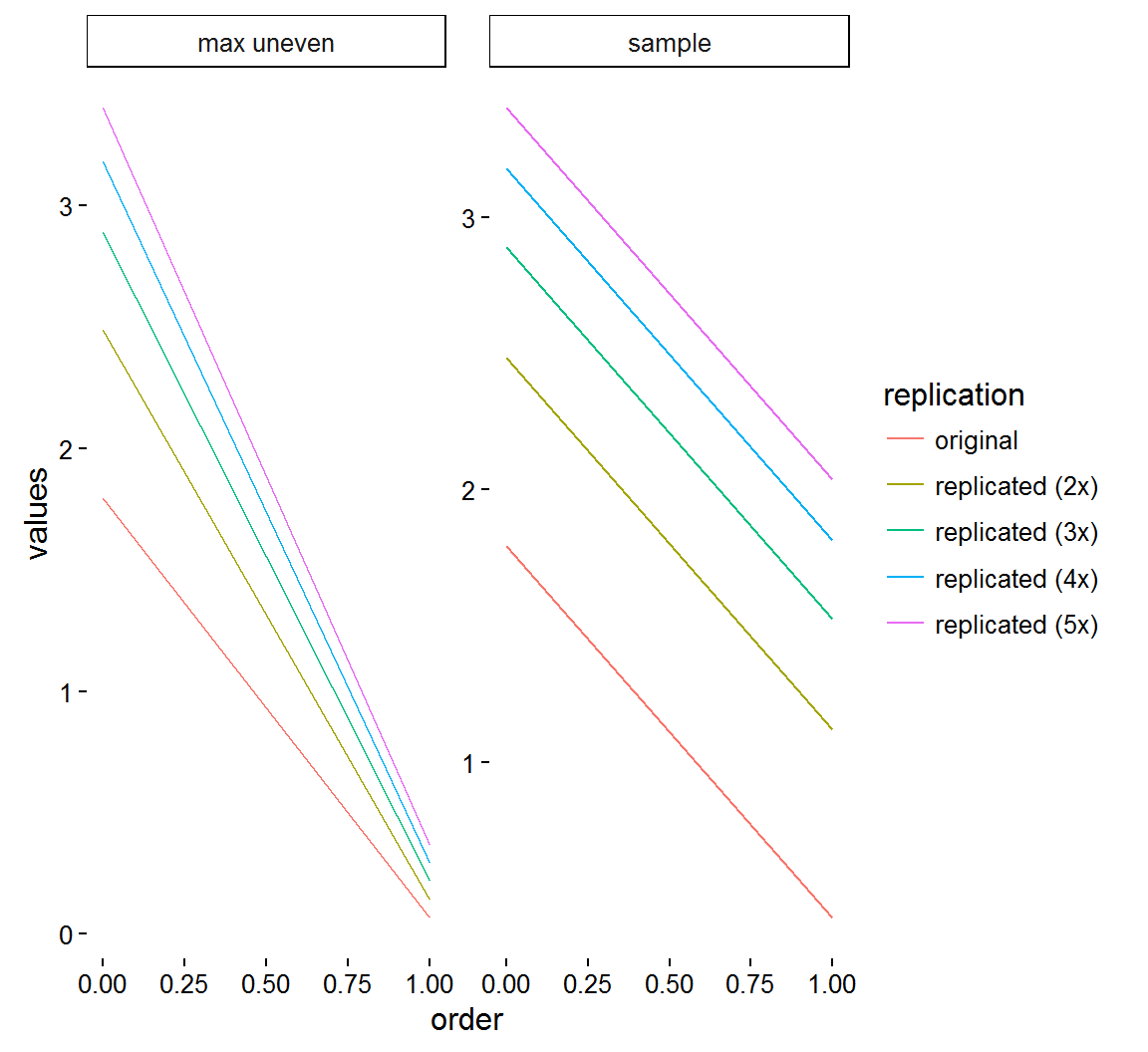• As seen in the plot, while the slope of the sample renyi specra does not change with replication, the slope of the equivalent maximally uneven populations does
• Since the $$RLI$$ is the ratio of the sample slope to the maximally unequal slope, it too is replication variant
• Since Pielou’s entropy is linearly related to $$RLI$$, it is also replication variant

Replication variance is a non-issue

• Pielou’s entropy (and thus, $$RLE$$ and $$RLI$$) was originally dismissed because it is not replication invariant
• However, replication invariance can conflict with intuition
• Compare Figure 1 populations in (Row B - Column 1) and (Row B - Column 2)
• (Row B - Column 1) is maximally uneven, so its relative evenness is zero
• (Row B - Column 2) could be considered a 2x replication of (Row B - Column 1)
• If evenness were replication in variant, then (Row B - Column 2) would also have a relative evenness of zero
• This is obviously a problem since (Row B - Column 2) is not maximally uneven

### The problem of sampling

• In a theoretical setting, we know all of the species in a population, regardless of how rare they are
• Realistically, population sampling will often miss rare species
• All of the measures of eveness discussed depend strongly on knowing true richness and small changes to this value can greatly alter the outcome
• Thus, the rare species missed in sampling can have a large impact on the apparent evenness of the population
• Example
pop <- c(100000,100000) #Perfectly even population of two species
pop.p1 <- c(pop, 1) #Addition of one rare species to population
pop.p2 <- c(pop.p1, 1)
pop.p3 <- c(pop.p2, 1)
pop.p4 <- c(pop.p3, 1)
pop.p5 <- c(pop.p4, 1)
pop.p6 <- c(pop.p5, 1)
pop.series <- list(pop, pop.p1, pop.p2, pop.p3, pop.p4, pop.p5, pop.p6)

RLE <- vector()
for (i in 1:7){
r <- renyi(pop.series[[i]], scales = c(0,1))
RLE <- c(RLE, r/r)
}

df <- data.frame("Additional species" = 0:6, "RLE" = RLE)
ggplot(data = df, aes(x = Additional.species, y = RLE)) + geom_line() + theme_classic()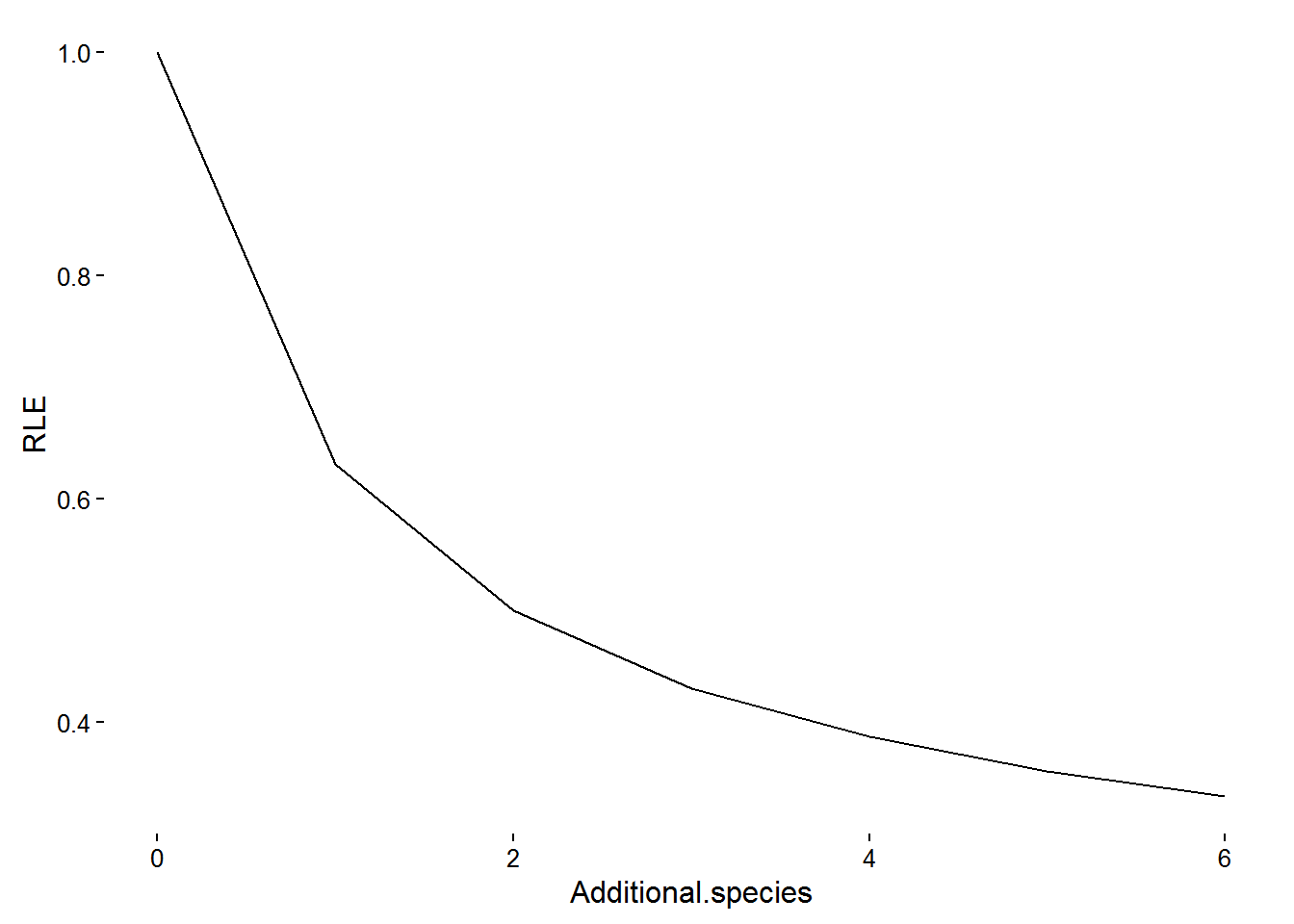Using higher order diversity

• The problem of sampling derives from needing to use $$D^0$$ to calculate $$RLE_{0,q}$$ and similar values
• However, an alternative approach would be to calculate $$RLE$$ with orders higher than zero, to avoid $$D^0$$ altogether + For $$RLE_{1,2}$$ this would simply be $$log(D^2)/log(D^1)$$
pop <- c(100000,100000) #Perfectly even population of two species
pop.p1 <- c(pop, 1) #Addition of one rare species to population
pop.p2 <- c(pop.p1, 1)
pop.p3 <- c(pop.p2, 1)
pop.p4 <- c(pop.p3, 1)
pop.p5 <- c(pop.p4, 1)
pop.p6 <- c(pop.p5, 1)
pop.series <- list(pop, pop.p1, pop.p2, pop.p3, pop.p4, pop.p5, pop.p6)

RLE0.1 <- vector()
RLE1.2 <- vector()
for (i in 1:7){
r <- renyi(pop.series[[i]], scales = c(0,1,2))
RLE0.1 <- c(RLE0.1, r/r)
RLE1.2 <- c(RLE1.2, r/r)
}

RLE <- c(RLE0.1, RLE1.2)

df <- data.frame("Additional species" = c(0:6, 0:6), "index" = c(rep("RLE0.1", 7), rep("RLE1.2",7)), "RLE" = RLE)

ggplot(data = df, aes(x = Additional.species, y = RLE, color = index)) + geom_line() + theme_classic()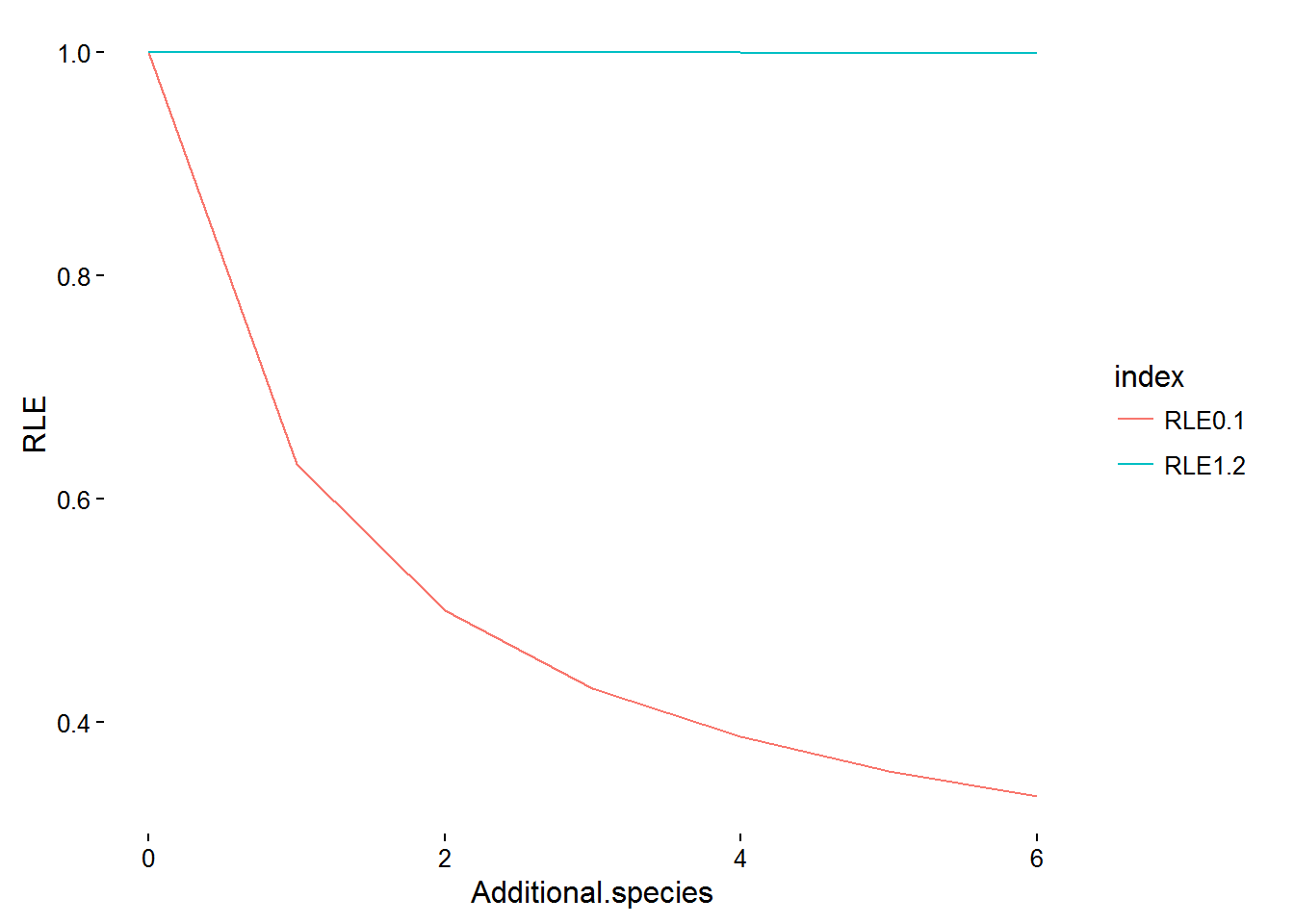* Notice that $$RLE_{1,2}$$ is essentially unaffected by the addition of rare species

Using improved measures of richness

• An alternative to avoiding $$D^0$$ woudl be to improve the estimation of richness
• Richness estimators
• Many non-parametric richness estimators (e.g. Chao) have been proposed
• However, they often provide only a lower bound of possible richness
• Cannot quantify the uncertainty of a richness estimator without parametric assumption
• Rarefication
• Rarefraction standardizes a population to a given standard size
• However, resampling does not preserve the replication principle
• If sample A has twice the richness of sample B, but both are rarefied to the same sample size, they will appear to have the same richness
• Coverage
• Coverage is the proportion of the individuals in a population represented by the species detected in a sample
• E.g. For a population of species frequencies $${A = 0.5, B = 0.3, C=0.18, D = 0.02}$$, if a sample obtains species A, B, and C, but not D, that sample would have 98% coverage of the population
• Cannot know “true” coverage without knowing true richness, but there are very good estimates
• Good’s coverage $$\boxed{C = 1-f_1/N}$$
• $$f_1$$ - singleton - a species that only has one abundance count
• $$N$$ - the total number of individuals (total abundance)
• The fewer singletons that are detected, the more likely full coverage has been reached
• A populaiton can be resampled to a given coverage to make fair evenness analyses